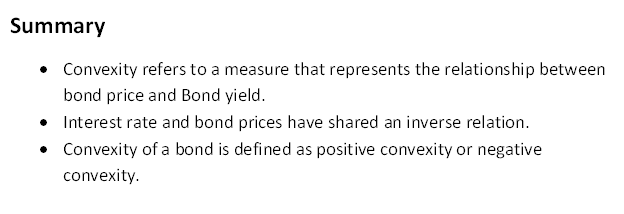# Convexity

What is Convexity?

Convexity refers to a measure that represents the correlation between bond price and Bond yield. It is used as a tool of risk management to manage and measure an exposure of a portfolio.Understanding Convexity

Convexity is used to measure the changes in bond price with the change in the interest rate. It is counted as a good measure of bond’s duration with a change in yield. It shows the change in duration of bond with the change in the interest rate.

Talking about convexity in mathematical terms, convexity is the first derivate of duration equation and second derivate formula which states the fluctuation in bond prices by a change in interest rates. Bond prices depend on various factors including interest rate. Change in yield will result change in its duration of bond too, convexity of bond measures the affectability of a bond’s duration with the change in yield.  The bond yield refers to an investor’s expected return or earning from purchasing or holding a particular security.

Convexity and duration of a bond have inverse relationship. Bond price will increase with the fall in interest rate. On the same side, bond price will fall with the rise in the interest rate. It helps an investor by providing a sight into how the price of a bond is affected by the fluctuation of interest rate in market. In other words, change in the duration of a bond with the change in yields will be measured by convexity.

What is bond duration in convexity?

Bond duration is a measure that’s shows the change in a price of bond’s with the change in the market interest rate. It also gives an idea how fluctuation of interest rate affect the price of a bond. A higher duration bond indicates the price of bond will move to a greater price in the reverse direction of interest rates. And same lower duration indicates the low price sensitivity, will move less with the change in interest. Duration helps in measuring the bond’s sensitivity with the fluctuation in interest rate.

The bond’s duration is affected by several factors including yield, coupon rate and maturity. If we are comparing two bonds it includes different scenarios are as follow:

• In a case of coupon and yield are the same, duration will increases with time left to maturity.
• In a case of maturity and yield are the same, duration will increases with fall in coupon.
• In a case of coupon and maturity are the same, duration will increases with fall in yield.

How do we define negative or positive Convexity?

Convexity of a bond is defined as positive convexity or negative convexity. If the duration of a bond increases with the decline in yield, a bond may have positive convexity. Likewise, if the duration of a bond moves up with the rise in yield, a bond may have negative convexity.

Positive convexity is working as an advantage for investors because prices of a bond become less sensitive with the fluctuation of yield, when the yield increase price will fall and if the yield fall the price will increase. A positive convexity bond prices are increased with more rate due to fall in yield than price fall with the rise in yield.

A negative convexity bond, prices of a bond will fall with high rate with an increase in yield. Therefore, if a bond has negative convexity, it indicates the duration rises with the fall in price and vice versa. A negative convexity worked against interest of an investor.

Convexity can be positive or negative. A positive convexity bond indicates the positive correlation between the duration and yield i.e. the duration of a bond and yield fluctuate together. A yield curve for positive convexity moves upward. This type of bond may not have a prepayment option or call option.

Negative convexity has a negative correlation between duration of bond and yield, i.e. when the yield increases, the duration will decrease. The yield curve for negative convexity moves downward. This type of bond may have call options, repayment option and have option of mortgage-backed securities.

How are Interest Rates and Bond Prices are related?

Talking about the relationship between interest rate and bond price, they shared inverse relation. The interest rate and the price of bond moves inversely, price of a bond increases with the fall in interest rate and will decrease with the rise in interest rate.

Interest rate is the common risk for all investors of bond as rise in interest rate leads decline in the price of bonds and fall in interest rate results increase in the price of bond.

Understand by taking an example, let assume the market rates (interest rates) are expected to raise, a bond holder willing to sell his existing bonds and looking for new bonds with a motive of enjoying more profit. But rise in interest rate results the fall in the price of bond. Here before investing in any bond, he/she will use convexity that shows the effect of change in bond price with the fluctuation in the market interest rate.

Convexity is a measure which helps to know the how duration of bond affects with the change in yields. This measurement helps an investor to understand how a bond is expected to perform with the change in interest rate, and provide sight into the price of the securities with the different interest rates in the market.

##### Top ASX Listed Companies

Definition:
We use cookies to ensure that we give you the best experience on our website. If you continue to use this site we will assume that you are happy with it. OK